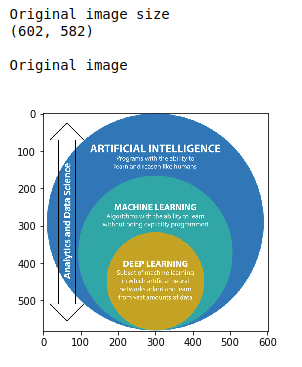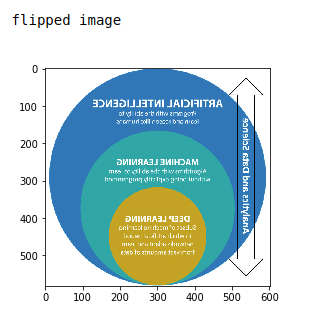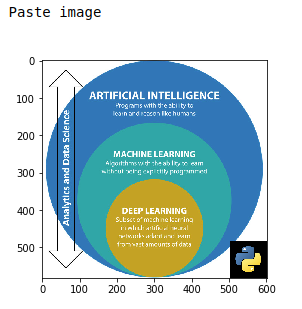• #5, First Floor, 4th Street Dr. Subbarayan Nagar Kodambakkam, Chennai-600 024 Landmark : Samiyar Madam
• pro@slogix.in
• +91- 81240 01111

### How to flip and paste an image using pillow library in python?

###### Description

To write a piece of python code to resize and crop an image using pillow library in python.

#### Input

JPG file. (Image file)

#### Output

Resized and cropped image.

###### Process

Import pillow library.

Call the constructor (transpose()) from pillow library.

Define size of the image.

Plot the flipped image using matplot.lib.

Call the paste constructor.

Plot the pasted image.

###### Sample Code

#import pillow library
from PIL import Image
import matplotlib.pyplot as plt

image = Image.open(‘ai-ml-dl.jpg’)
print(“Original image size”)
print(image.size,”\n”)
print(“Original image\n”)
plt.imshow(image)
plt.show()

#flip the image
print(“flipped image\n”)
image_flip = image.transpose(Image.FLIP_LEFT_RIGHT)
image_flip.save(‘image_flip.jpg’)
plt.imshow(image_flip)
plt.show()

#cropping the image
print(“Paste image\n”)
logo = Image.open(‘logo.png’)
logo = logo.resize((100,100))
image_copy = image.copy()
position = ((image_copy.width – logo.width), (image_copy.height – logo.height))
image_copy.paste(logo, position)
image_copy.save(‘pasted_image.jpg’)
plt.imshow(image_copy)
plt.show()

###### Screenshots